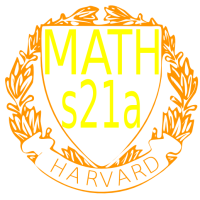Course 30189: Maths21A Summer 2008
Multivariable Calculus
FAQ
Office: SciCtr 434
Send questions of potential general interest to math21b@fas.harvard.edu.

 Question:In the first homework, there are relations in space which do not involve the z variable. Does this define an object in the plane? Answer: Question:No, in problem 1, all sets are sets in space. If you take for example the set x22 + z2 = 1, then the y variable is not involved. So, we can chose y arbitrarily. The equation defines a cylinder in space. Answer: Is it ok to use an older edition of a textbook.